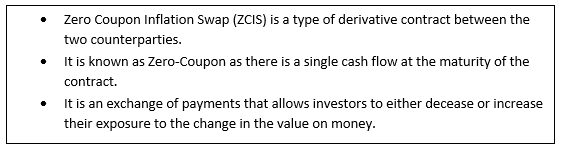# Zero Coupon Inflation Swap

• Updated on

What is Zero Coupon Inflation Swap (ZCIS)?

Zero Coupon Inflation Swap (ZCIS) is a type of derivative contract between the two counterparties in which a fixed-rate payment is exchanged for a payment at the rate of inflation. The process involves one party which makes payments based on inflation floating rate, while other makes payment based on fixed swap rate.

In case, if the inflation rate increases the inflation buyer receive higher from the inflation seller as compared to what they paid and if the inflation rate falls, the inflation buyer gets less from the inflation seller as compared to what they paid.With a Zero Coupon Inflation Swap, when the swap contract reaches its maturity and the inflation rate is known rate both income streams are paid as a single lump sum amount, instead of exchanging periodic payments.

It is known that Zero-Coupon is a single cash flow at the maturity of the contract, without any intermediate coupon and the final single cash flow is the difference between the value of the floating amount and the fixed amount at maturity.

It is an exchange of payments that allows investors to either decease or increase their exposure to the change in the value on money. Zero Coupon Inflation Swap is also known as breakeven inflation swap or breakeven swap.

Understanding a Zero-Coupon Inflation Swap

With this procedure, the inflation risk is passed on from one party to another through an exchange of fixed cash flow. It is a kind of inflation derivative in which a cash flow with the rate of inflation is exchanged for a cash flow with a fixed interest rate.

A Zero-coupon security, during the life of the investment does not make any periodic interest payment. Instead, a security holder gets a lump sum payment on the maturity date.

Similarly, when Zero Coupon Inflation swaps reach maturity and the inflation level are known, both the cash flows are paid as one lump-sum payment that depends on the inflation rate reached over a specific time duration. Zero Coupon Inflation Swap is a bilateral contract that is used to provide a hedge against inflation.

As the payment is made at the maturity, a buyer may sell the swap contract on the over-the-counter (OTC) market prior to maturity.

Under a Zero-Coupon Inflation swap, the inflation buyer or receiver pays a pre-agreed fixed rate, which is referred to as fixed leg and receives an inflation linked payment from the inflation seller or payer, which is referred as inflation leg. The fixed rate is known as the breakeven swap rate.

The payment from both the fixed and inflation legs represents the difference between expected and actual inflation. If the actual inflation is higher than the expected inflation, the return to the buyer is considered as a capital gain. As inflation increases, the buyer earns more and vice-versa.

How to calculate Zero Coupon Inflation Swap

The inflation receiver pays a fixed amount known as the fixed leg. This is:

Fixed Cash Flow/ Fixed Leg = A x [(1+r)t -1]

The inflation payer pays an amount that is given by the change in the inflation index, known as the inflation leg. This is:

Floating Cash Flow/ Inflation Leg = A x [(IM/II) -1]

Where:

A= Notional amount

r= Fixed swap rate

t= Time in years

IM= Inflation index level at maturity

II= Inflation index level at initiation

In the beginning of the contract both the parties needs to agree on the terms and predetermine maturity date, fixed rate to be exchanged and choose a notional amount. Notional amount is the principal amount from which cash flows are calculated but not exchanged.  Bothe the parties can also put up collateral to prevent counterparty default risk.

Unlike other derivatives contract traded on an exchange, swaps are traded on the over-the-counter (OTC), which means parties can change any specific details to suit their needs. The contract may be ended before the maturity date by calculating the market value and buying out the counterparty. Initially the value of the contract is at par as the terms are based on the current market conditions at the time and with the time the inflation rate changes so the market value also changes positively or negatively.

Example

Two parties enter into a five-year Zero coupon inflation swap with a notional amount of \$100 million, a 2.4% fixed rate and the agreed-upon inflation index at 2.0%. At maturity, the inflation index is at 2.5%.

Fixed Cash Flow = \$100,000,000 * [(1.024)5 – 1)] = \$100,000,000 * [1.1258999 – 1]

= \$12,589,990.68

Floating Cash Flow = \$100,000,000 * [(0.025 ÷ 0.020) – 1] = \$100,000,000 * [1.25 – 1]

= \$25,000,000.00

Since the compounded inflation rate rose above 2.4%, inflation buyer receives higher from the inflation seller as compare to what they paid.

We use cookies to ensure that we give you the best experience on our website. If you continue to use this site we will assume that you are happy with it. OK